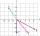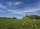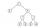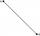# Unit vector 2D

Determine coordinates of unit vector to vector AB if A[-6; 8], B[-18; 10].

x =  -0.99
y =  0.16

### Step-by-step explanation:

$y=\frac{\mathrm{\Delta }y}{\mathrm{\mid }\text{\vect}AB\mathrm{\mid }}=0.16$Did you find an error or inaccuracy? Feel free to write us. Thank you!

Showing 1 comment:Tza0987
097=134
134=824
824=650
650=?Tips to related online calculators
Line slope calculator is helpful for basic calculations in analytic geometry. The coordinates of two points in the plane calculate slope, normal and parametric line equation(s), slope, directional angle, direction vector, the length of the segment, intersections of the coordinate axes, etc.
Our vector sum calculator can add two vectors given by their magnitudes and by included angle.
Pythagorean theorem is the base for the right triangle calculator.

#### You need to know the following knowledge to solve this word math problem:

We encourage you to watch this tutorial video on this math problem:

## Related math problems and questions:

• Vectors abs sum diffThe vectors a = (4,2), b = (- 2,1) are given. Calculate: a) |a+b|, b) |a|+|b|, c) |a-b|, d) |a|-|b|.
• VectorsVector a has coordinates (8; 10) and vector b has coordinates (0; 17). If the vector c = b - a, what is the magnitude of the vector c?
• Calculate 6Calculate the distance of a point A[0, 2] from a line passing through points B[9, 5] and C[1, -1].
• Distance problem 2A=(x,2x) B=(2x,1) Distance AB=√2, find value of x
• VectorCalculate length of the vector v⃗ = (9.75, 6.75, -6.5, -3.75, 2).
• ExpressionsFind out value of expressions if a = -1, b =2: x=b - 2a - ab y=a3 - b2 - 2ab z=a2 b3 - a3 b2 w=a + b + a3 - b2
• Three pointsThree points A (-3;-5) B (9;-10) and C (2;k) . AB=AC What is value of k?
• SegmentCalculate the segment AB's length if the coordinates of the end vertices are A[10, -4] and B[5, 5].
• A Cartesian framework1. In a Cartesian framework, the functions f and g we know that: the function (f) is defined by f (x) = 2x ^ 2, the function (g) is defined by g (x) = x + 3, the point (O) is the origin of the reference, point (C) is the point of intersection of the graph
• Vector 7Given vector OA(12,16) and vector OB(4,1). Find vector AB and vector |A|.
• Triangle IRTAn isosceles right triangle ABC with right angle at vertex C has vertex coordinates: A (-1, 2); C (-5, -2) Calculate the length of segment AB.
• SquarePoints A[9,9] and B[-4,1] are adjacent vertices of the square ABCD. Calculate the area of the square ABCD.
• Three points 2The three points A(3, 8), B(6, 2) and C(10, 2). The point D is such that the line DA is perpendicular to AB, and DC is parallel to AB. Calculate the coordinates of D.
• 3d vector componentThe vector u = (3.9, u3) and the length of the vector u is 12. What is is u3?
• Vector - basic operationsThere are given points A [-9; -2] B [2; 16] C [16; -2] and D [12; 18] a. Determine the coordinates of the vectors u=AB v=CD s=DB b. Calculate the sum of the vectors u + v c. Calculate difference of vectors u-v d. Determine the coordinates of the vector w
• DistanceWhat is the distance between the origin and the point (18; 22)?
• Angle of the body diagonalsUsing vector dot product calculate the angle of the body diagonals of the cube.